# The Mole MgH Lab Valence electrons MgH Lab

• Slides: 22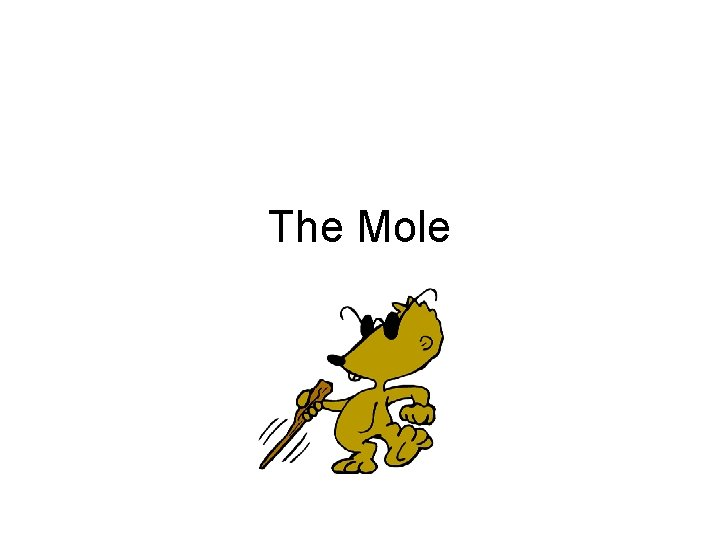The Mole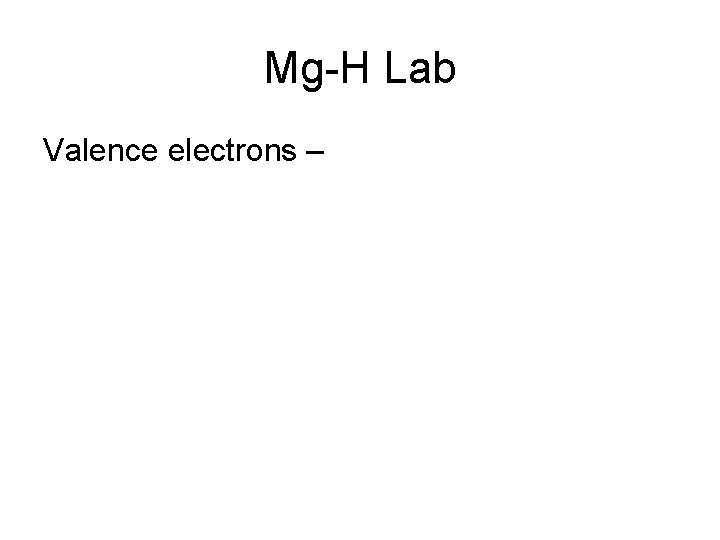Mg-H Lab Valence electrons –Mg-H Lab Valence electrons - electrons in outer most orbit. Involved in chemical reactions.Mg-H Lab Valence electrons – electrons in outer most orbit. Involved in chemical reactions.Mg-H Lab • Need to be able to count atoms.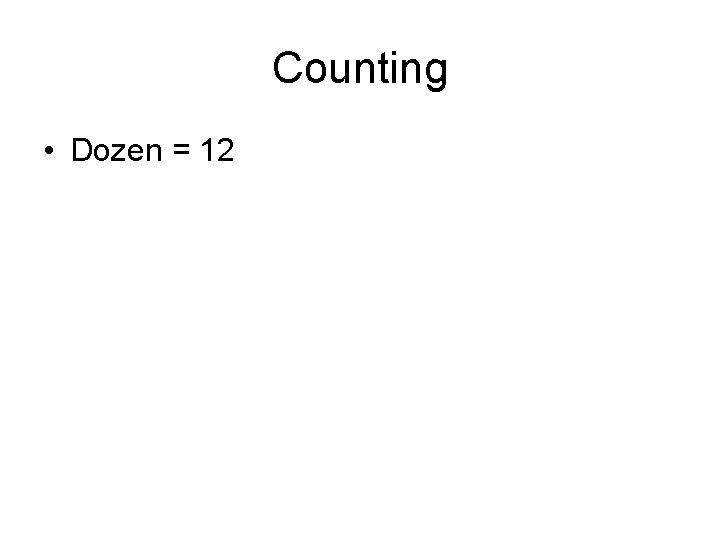Counting • Dozen = 12Counting • Dozen = 12 • Ream =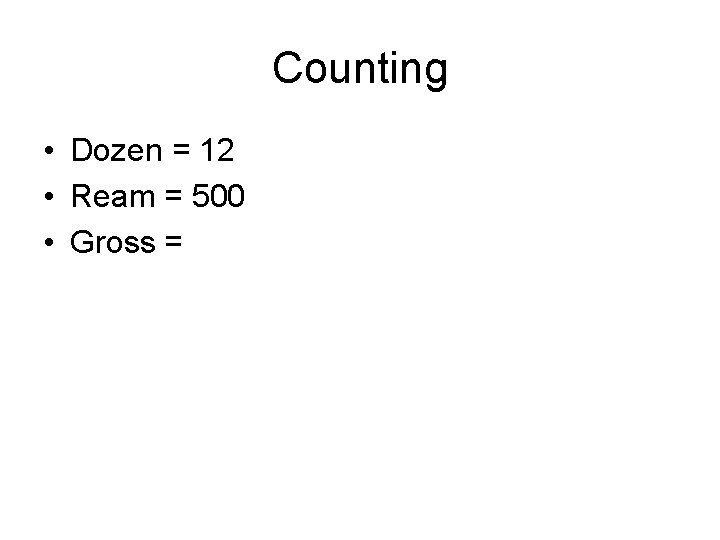Counting • Dozen = 12 • Ream = 500 • Gross =Counting • Dozen = 12 • Ream = 500 • Gross = 144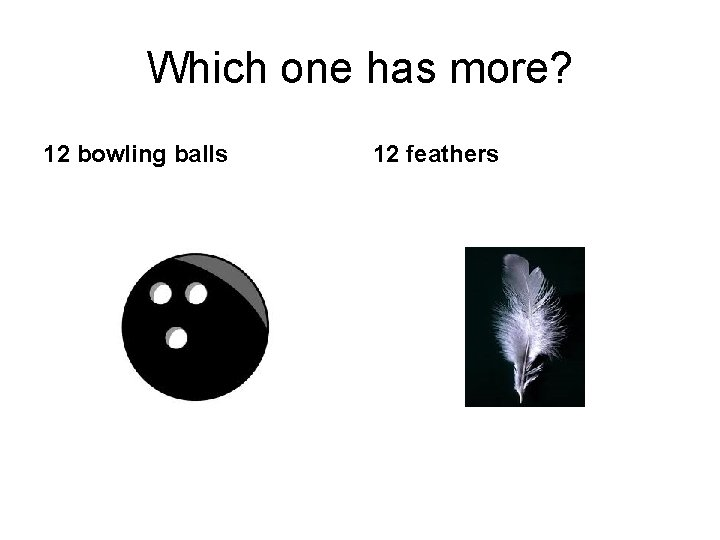Which one has more? 12 bowling balls 12 feathers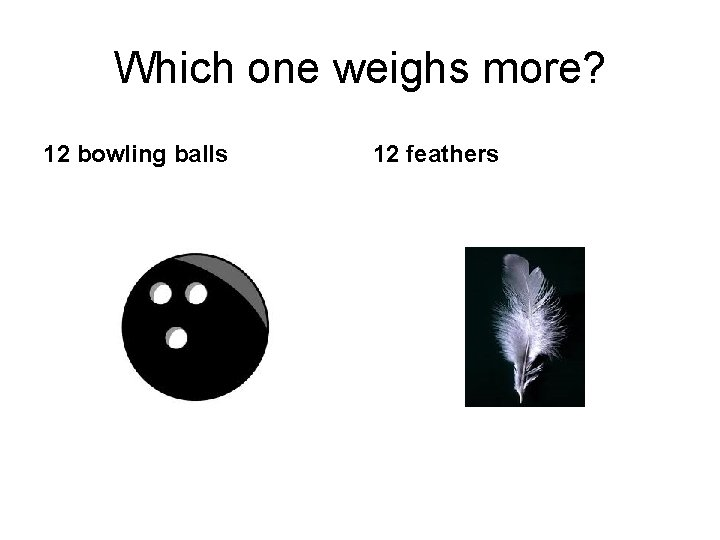Which one weighs more? 12 bowling balls 12 feathersThe Mole • Mole is a way to count atoms • 1 mole = 6 x 1023 • 1 mole of pennies = 6 x 1023 pennies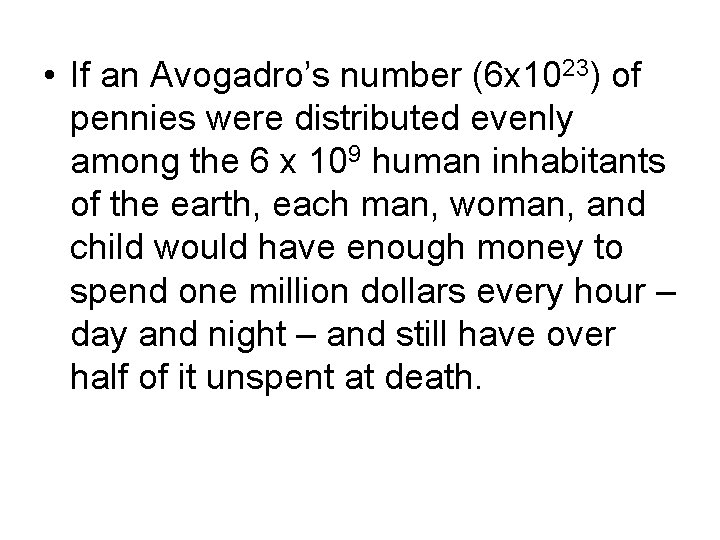• If an Avogadro’s number (6 x 1023) of pennies were distributed evenly among the 6 x 109 human inhabitants of the earth, each man, woman, and child would have enough money to spend one million dollars every hour – day and night – and still have over half of it unspent at death.• If you were to try to count the number of atoms in exactly 12 grams of carbon, and proceed at the rate of 100 atoms per minute without interruption, 24 hours per day, 365 days per year, the task would require……. .• If you were to try to count the number of atoms in exactly 12 grams of carbon, and proceed at the rate of 100 atoms per minute without interruption, 24 hours per day, 365 days per year, the task would require……. . • 11, 500, 000, 000 years.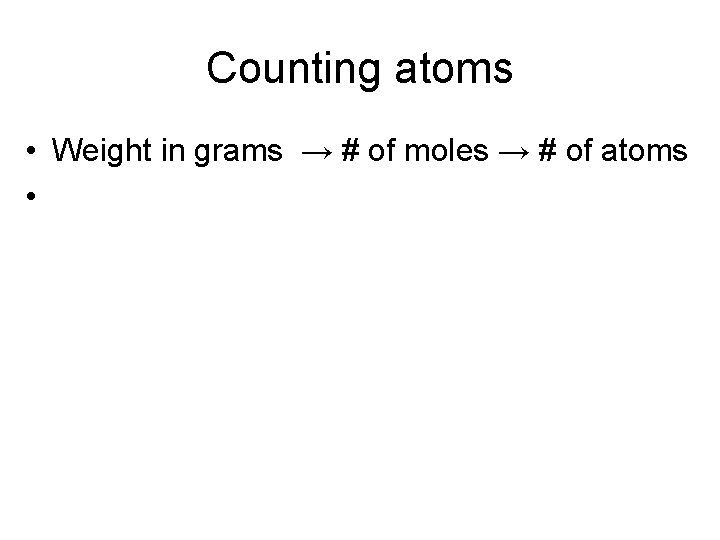Counting atoms • Weight in grams → # of moles → # of atoms •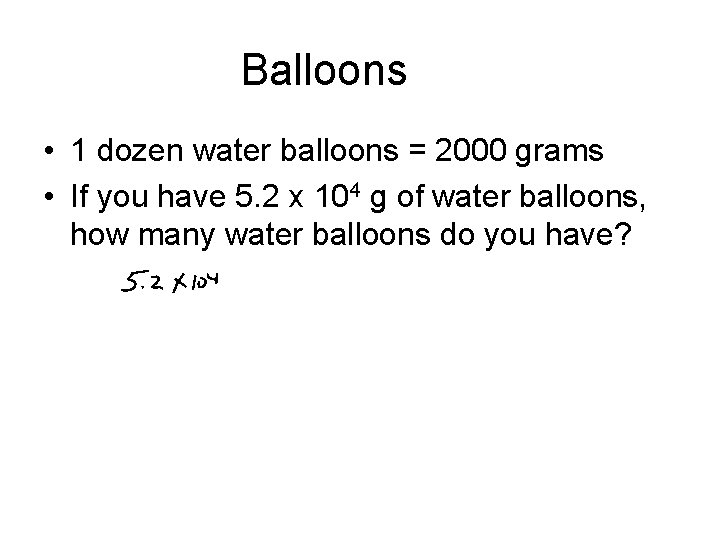Balloons • 1 dozen water balloons = 2000 grams • If you have 5. 2 x 104 g of water balloons, how many water balloons do you have?• 1 dozen = 12 • 1 mole = 6 x 1023 • 1 mole of any element = 6 x 1023 atoms • 1 mole of any element = atomic mass in grams of that element• 1 mole of Aluminum = ____ grams • 1 mole of Iron = ____ grams • 1 mole of Sulfur = _____ gramsHow many moles of Mg? • If we have 0. 5 g of Magnesium, how many moles do we have?How many atoms of Mg? • If we have 0. 5 g of Magnesium, how many atoms do we have?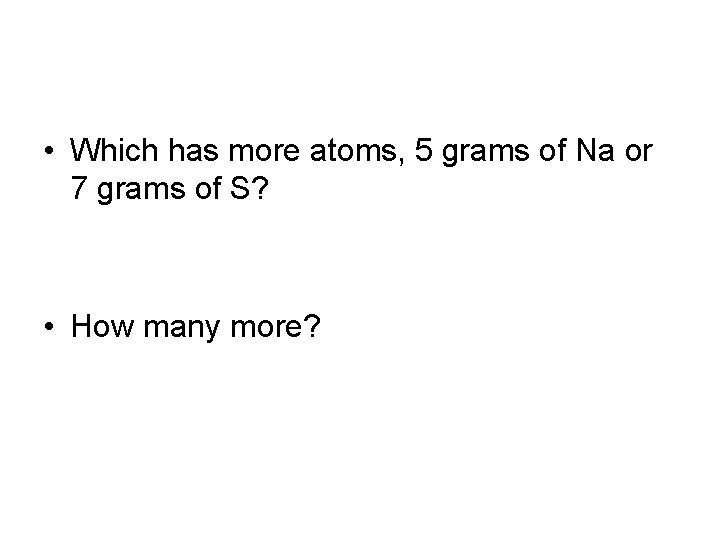• Which has more atoms, 5 grams of Na or 7 grams of S? • How many more?